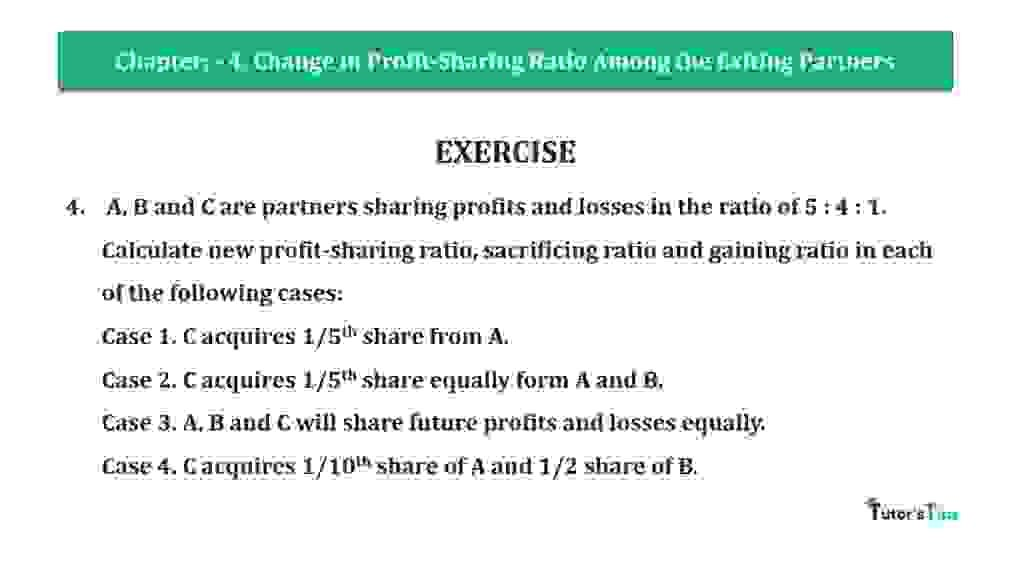# Question 04 Chapter 4 of +2-A – T.S. Grewal 12 Class Part – A Vol. 1Question 04 Chapter 4 of +2-A

4. A, B and C are partners sharing profits and losses in the ratio of 5 : 4 : 1.
Calculate new profit-sharing ratio, sacrificing ratio and gaining ratio in each of the following cases:
Case 1. C acquires 1/5th share from A.
Case 2. C acquires 1/5th share equally form A and B.
Case 3. A, B and C will share future profits and losses equally.
Case 4. C acquires 1/10th share of A and 1/2 share of B.

The solution of Question 04 Chapter 4 of +2-A

:

Case I C acquires 1/5th from A

 Old Ratio of A,B, & C = 5 : 4 : 1

A sacrifice 1/5th share of Profit
C gain 1/5th share of Profit
Calculate the A, B, & C New Profit sharing ratio: –
Partner’s New Profit share = Old Ratio − or + Sacrifice/gain

 A’s New Share = 5 – 1 10 5
 = 15 -2 10
 = 3 30
 B’s New Share = 4 No change 10
 C’s New Share = 1 – 1 10 5
 = 6 – 2 10
 = 3 10

New Profit Ratio of A,B, & C = 3 : 4 : 3

Case II C acquires 1/5th share equally form A and B.

Old Ratio of A,B, & C = 5 : 4 : 1
A sacrifice 1/10th share of Profit
A sacrifice 1/10th share of Profit
C gain 1/5th share of Profit
Calculate the A, B, & C New Profit sharing ratio: –
Partner’s New Profit share = Old Ratio − or + Sacrifice/gain

 A’s New Share = 5 – 1 10 10
 = 5 -1 10
 = 4 10
 B’s New Share = 4 – 1 10 10
 = 4 -1 10
 = 3 10
 B’s New Share = 1 + 1 + 1 10 10 10
 = 1+1+1 10
 = 3 10

New Profit Ratio of A,B, & C = 4 : 3 : 3

Case III A, B and C will share future profits and losses equally.

Old Ratio of A, B, & C = 5 : 4 : 1
New Ratio of A, B, & C = 1 : 1 : 1
Calculate the Sacrificing or Gaining Ratio of Partners
Sacrificing or Gaining Ratio= Old Ratio – New Ratio

 A’s New Share = 5 – 1 10 3
 = 15 -10 30
 = 5 Sacrificing 10
 B’s Share Sacrificing/Gaining = 4 – 1 10 3
 = 12 -10 30
 = 2 Sacrificing 10
 B’s Share Sacrificing/Gaining = 1 – 1 10 3
 = 3-10 30
 = – 7 Gaining 10

 Sacrificing Share of A = 5 30
 Sacrificing Share of B = 2 30
 Gaining Share of C = -7 30

Case IV C acquires 1/10th share of A and 1/2 share of B.

Old Ratio of A,B, & C= 5 : 4 : 1
A sacrifice 1/10th of his share
A sacrifice 1/2th of his share
Calculate the A, B, & C New Profit sharing ratio: –
Partner’s Sacrifice fixed portion of him share = Old Ratio X Sacrificed portion

 A’s New Share = 5 X 1 10 3
 = 1 Sacrificing 20
 B’s Sacrifice to C = 4 X 1 10 3
 = 4 Sacrificing 20
 B’s Sacrifice to C = 4 + 1 20 20
 = 1 + 4 30
 = 5 Gaining 20

Partner’s New Profit share = Old Ratio − or + Sacrifice/gain

 A’s New Share = 5 – 1 10 20
 = 10 – 4 20
 = 9 20
 B’s New Share = 4 – 4 10 20
 = 8 – 4 20
 = 4 20
 C’s New Share = 1 + 1 + 4 10 20 20
 = 2 + 1 + 4 20
 = 7 20

New Profit Ratio of A, B, & C = 9 : 4 : 7

### T.S. Grewal’s Double Entry Book Keeping +2 (Vol. I: Accounting for Not-for-Profit Organizations and Partnership Firms)

• Chapter No. 1 – Financial Statement of Not-For-Profit Organisations
• Chapter No. 2 – Accounting for Partnership Firms – Fundamentals
• Chapter No. 3 – Goodwill: Nature and Valuation
• Chapter No. 4 – Change in Profit-Sharing Ratio Among the Existing Partners
• Chapter No. 5 – Admission of a Partner
• Chapter No. 6 – Retirement/Death of a Partner
• Chapter No. 7 – Dissolution of a Partnership Firm

### T.S. Grewal’s Double Entry Book Keeping (Vol. II: Accounting for Companies)

• Chapter No. 1 – Financial Statements of a Company
• Chapter No. 2 – Financial Statement Analysis
• Chapter No. 3 – Tools of Financial Statement Analysis – Comparative Statements and Common- Size Statements
• Chapter No. 4 – Accounting Ratios
• Chapter No. 5 – Cash Flow StatementVol. I: Accounting for Not-for-Profit Organizations and Partnership Firms